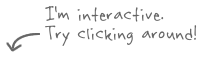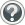Membean is an incredibly effective way to learn words and permanently remember them.
Learn more on how we help for Test Prep, Personal Learning, or get it for your School.

archives »# #124 equ → equal

## Quick SummaryThe Latin root word equ means “equal.” This Latin root is the word origin of a good number of English vocabulary words, including adequate, equator, and equality. The Latin root word equ is easily recalled through the word equation, for both sides of an equation are, by definition, “equal” to one another!

## From Membean

The word ingredient Memlet, shown below, is one of many ways that a word is taught in Membean.
See an example word page »

### Ingredient Memlet: equanimityequ → equal anim → mind, spirit -ity → state or quality

Equanimity is the “state of equal mind,” that is, one’s mind does not become agitated but rather stays even and peaceful.

### Ingredient Memlet: adequatead- → to, towards equ → equal -ate → possessing a certain quality

An adequate amount of something is “equal to or towards” what is needed.

## All Words Created with “Equ”

The Latin root word equ means “equal.” Soon enough you will learn to equate equ with “equal”—especially since the meaning of the root equ, “equal,” contains the root itself!

Math classes are filled with words that have the Latin root word equ in them. Math teachers talk about equations all the time, which are expressions like x = 2y or y = mx + b, where both sides of the equation are “equal” to one another. Indeed, if x = y – 2, then you can equate x with a number that is 2 less than y, which means that x “equals” y – 2. Geometry teachers love to talk about equilateral triangles, whose three sides are “equal” in length to one another. This makes the measurement of each side equivalent, or “equal” in value to each other.

Not to be outdone by math teachers, teachers of geography have terms with equ meaning “equal” in them as well. Consider the nearly 25,000-mile line of latitude, the equator, which is of “equal” distance to or from each pole, thereby dividing the Earth into “equal” halves. The vernal (spring) and autumnal (fall) equinoxes fall on days when there are an “equal” number of hours of daylight and darkness due to the fact that the sun is standing right over the equator.

Government teachers often chat about equality of humans, meaning that all humans are created “equal.” Most governments, unfortunately, do not do an adequate job, or “equal” to what is needed, when it comes to treating all of its citizens “equally.” Imagine if all humans were indeed treated equally—what equanimity would arise from that! Equanimity is a state of “equal” mind, that is, a mind that is not agitated or imbalanced but rather is even and therefore peaceful. Then the world would be more in a state of equilibrium, or “equally” balanced so that no one would feel mistreated—how great would that be?

You should now feel “equal” to the task of recognizing the root word equ, giving you a pleasant state of linguistic equanimity!

1. equal: of being the same, or “equal”
2. equation: condition of two sides of a mathematical expression being “equal” to one another
3. equate: to make two things “equal” to one another
4. equilateral: having sides that are “equal”
5. equivalent: having “equal” value
6. equator: line of latitude which divides planet Earth into “equal” halves
7. equinox: a time of “equal” light and darkness
8. equality: state or condition of being “equal”
9. adequate: “equal” to what is needed
10. equanimity: a state of the mind being “equal” or peaceful
11. equilibrium: a state of being “equally” or evenly balanced